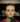# Beginning Thoughts on The Abstract Algorithm

March 28, 2019

Warnings: Haskell knowledge required, oversimplifications of Haskell evaluation strategy within

The Abstract Algorithm is an attempt to avoid computing the same data multiple times. Imagine you have the following Haskell function:

``doubleFirst x y = x + x ``

Clearly, not a very useful function. It just doubles `x`, and completely ignores `y`. Now, let’s say we pass it the following values.

``i = doubleFirst (3*3*3) (4^10)``

There are two obvious ways to actually call `doubleFirst`, or more accurately, “reduce the definition of `i`”. The first is by replacing the arguments with the parameters:

``````doubleFirst x y = x + x
i = doubleFirst (3*3*3) (4^10)
-- becomes...
i = (3*3*3) + (3*3*3)
-- becomes...
i = 54``````

This is clearly not optimal - we will end up doing the `3*3*3` calculation twice. Instead, let’s try evaluating before we replace the arguments with the parameters.

``````doubleFirst x y = x + x
i = doubleFirst (3*3*3) (4^10)
-- becomes...
i = doubleFirst 27 1048576
-- becomes...
i = 27 + 27
-- becomes...
i = 54``````

This is no better! Since we now evaluate before we substitute, we end up evaluating `4^10` when we didn’t have to.

Haskell solves this issue the first way, with what they call lazy evaluation, or non-strict evaluation. Most (all?) imperative languages solve this the second way, called eager or strict evaluation. Now, haskell is a bit smarter than this because it will attempt to check when you’ve reused a value and not calculate it twice, but it’s still not perfect.

Some quick terminology:

1. Expression: Something like `27 + 27` or `5` or `doubleFirst 10 20`.

2. Reduce/reduction: Converting an expression like `doubleFirst (3*3*3) (4^10)` to a simpler form, like `doubleFirst 27 1048576` or `(3*3*3) + (3*3*3)`.

3. Normal form: When it’s impossible to reduce an expression any further, like `54`.

4. Redex: An expression which is possible to reduce. `27 + 27` is a redex because you can reduce it to `54`, but `54` is not a redex because it is not in normal form.

5. Reduction strategy: A way of going about reducing expressions, ideally to normal form. Lazy and Eager evaluation are both reduction strategies.

The Abstract Algorithm provides a way to solve this issue that has 2 very desirable properties. For example, it can reduce an expression to normal form using the same or fewer steps than any other general strategy. This is called Optimality. It also has the guarantee that if any reduction strategy could reduce an expression to normal form, then the Abstract Algorithm can. This is called Correctness. Remembering from earlier:

1. Lazy evaluation will reduce everything to normal form eventually, so it is Correct. However, it will not reduce it in the fewest possible number of steps because it will sometimes duplicate work, so it is not Optimal. In our example, this was when we had to evaluate `(3*3*3)` to `27` twice because we used it twice in the output.

2. Eager evaluation, on the other hand, will not reduce everything to normal form eventually. This means it is not Correct. An example of when an eagerly-evaluated program will never be made into normal form is the following:

``````x = y
y = x
doubleFirst 5 x``````

When trying to reduce this, lazy evaluation has no issues. It never tries to evaluate `x`, so its definition does not matter. But if you try to eagerly evaluate x, then you will see this:

``````x = y
y = x
doubleFirst 5 x
-- becomes...
doubleFirst 5 y
-- becomes...
doubleFirst 5 x
-- becomes...
doubleFirst 5 y
-- etc.``````

You may think that this is cheating, but the fact is that lazy evaluation can evaluate it and eager evaluation cannot. Aside from not being Correct, eager evaluation is also not Optimal, because it does the unnecessary work of calculating the second parameter to `doubleFirst` when `doubleFirst` completely ignores it.

The Abstract Algorithm is provably both Correct and Optimal. If this seems surprising, it should be. It took 10 years to develop and is yet beautifully simple and unlike anything else you’ve ever seen. Some people like to claim that Lisp is alien technology, and using it is like playing with the primordial forces of the universe. This may be true, but to me, the Abstract Algorithm is on another level entirely.

It also has some nice (but less important) features. It is quite easy to make parallel, so it’s possible to do reduction on the GPU, although the conversion to make it parallel may cause you to repeat some work. It is also fast. Absal, an implementation of the abstract algorithm, is capable of doing over 30 million rewrites/second (where a rewrite is roughly analogous to reducing an expression a tiny bit) and it does not do anything in parallel.

Note that normal form does not always exist. For example, consider the following function:

``````x = y
y = x
doubleFirst x 3 ``````

Here, there is no way to ever evaluate this function and bring it to normal form. In this case, you can say that reduction strategies diverge.

Unfortunately, there is a catch. The Abstract Algorithm technically works on something called “lambda calculus”, which is slightly different from haskell. Lambda calculus has a less-nice syntax than Haskell, but is otherwise extremely similar (lambda calculus also does not have types, while Haskell does).Written by Andre Popovitch, a Michigan State student who wants to change the world. You should follow him on Twitter